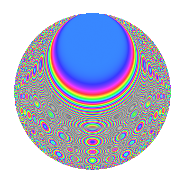# Properties

 Label 1008.2.bbLevel 1008 Weight 2 Character orbit bb Rep. character $$\chi_{1008}(125,\cdot)$$ Character field $$\Q(\zeta_{4})$$ Dimension 128 Sturm bound 384

# Related objects

## Defining parameters

 Level: $$N$$ = $$1008 = 2^{4} \cdot 3^{2} \cdot 7$$ Weight: $$k$$ = $$2$$ Character orbit: $$[\chi]$$ = 1008.bb (of order $$4$$ and degree $$2$$) Character conductor: $$\operatorname{cond}(\chi)$$ = $$336$$ Character field: $$\Q(i)$$ Sturm bound: $$384$$

## Dimensions

The following table gives the dimensions of various subspaces of $$M_{2}(1008, [\chi])$$.

Total New Old
Modular forms 400 128 272
Cusp forms 368 128 240
Eisenstein series 32 0 32

## Trace form

 $$128q + O(q^{10})$$ $$128q - 8q^{16} - 48q^{22} + 24q^{28} - 72q^{58} + 96q^{64} - 16q^{67} + 24q^{70} + 8q^{88} - 48q^{91} + O(q^{100})$$

## Decomposition of $$S_{2}^{\mathrm{new}}(1008, [\chi])$$ into newform subspaces

The newforms in this space have not yet been added to the LMFDB.

## Decomposition of $$S_{2}^{\mathrm{old}}(1008, [\chi])$$ into lower level spaces

$$S_{2}^{\mathrm{old}}(1008, [\chi]) \cong$$ $$S_{2}^{\mathrm{new}}(336, [\chi])$$$$^{\oplus 2}$$

## Hecke Characteristic Polynomials

There are no characteristic polynomials of Hecke operators in the database